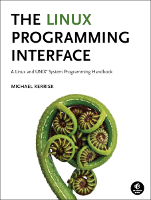This is threads/one_time_init.c, an example to accompany the book, The Linux Programming Interface.

This file is not printed in the book; it is the solution to Exercise 31-1 (page 670).

In the listing below, the names of Linux system calls and C library functions are hyperlinked to manual pages from the Linux man-pages project, and the names of functions implemented in the book are hyperlinked to the implementations of those functions.• Distribution version

Function list (Bold in this list means a function is not static)

/* one_time_init.c

The one_time_init() function implemented here performs the same task as
*/
#include "tlpi_hdr.h"

struct once_struct {            /* Our equivalent of pthread_once_t */
int called;
};

#define ONCE_INITIALIZER { PTHREAD_MUTEX_INITIALIZER, 0 }

struct once_struct once = ONCE_INITIALIZER;
static int
one_time_init(struct once_struct *once_control, void (*init)(void))
{
int s;

if (s == -1)

if (!once_control->called) {
(*init)();
once_control->called = 1;
}

if (s == -1)

return 0;
}
/* Remaining code is for testing one_time_init() */

static void
init_func()
{
/* We should see this message only once, no matter how many
times one_time_init() is called */

printf("Called init_func()\n");
}
static void *
{
/* The following allows us to verify that even if a single thread calls
one_time_init() multiple times, init_func() is called only once */

one_time_init(&once, init_func);
one_time_init(&once, init_func);
return NULL;
}
int
main(int argc, char *argv[])
{
int s;

/* Create two threads, both of which will call one_time_init() */

if (s != 0)

if (s != 0)

if (s != 0)